# NCERT Solution Exercise 7.3 class 10

Q.1 Find the area of the triangle whose vertices are :

(i) (2,3),(-1,0),(2,-4)

(ii) (-5,-1),(3,-5),(5,2)

Solution:

(i) (2,3),(-1,0),(2,-4)

Let The co-ordinate of vertices of the triangle are A  (2, 3), B (-1, 0), C(2, -4)

x1 = 2 ; y1 = 3

x2 = -1 ; y2 = 0

x3 = 2 ; y3 = -4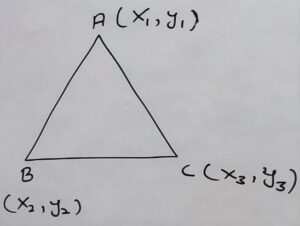Area of Δ ABC = 1/2 [ x1 (y2 – y3)  + x2 (y3 – y1) + x3 (y1 – y2) ]

⇒ Area of Δ ABC = 1/2 [ 2 ( 0 + 4 ) + (-1) (-4 – 3) + 2 (3 – 0) ]

⇒ Area of Δ ABC = 1/2 [ 2 ( 4 ) + (-1) (- 7) + 2 (3 ) ]

⇒ Area of Δ ABC = 1/2 [ 8 + 7 + 6 ]

⇒ Area of Δ ABC = 1/2 

⇒ Area of Δ ABC = 21/2 sq. units

(ii) (-5,-1),(3,-5),(5,2)

x1 = -5 ; y1 = -1

x2 = 3 ; y2 = -5

x3 = 5 ; y3 = 2Area of Δ ABC = 1/2 [ x1 (y2 – y3)  + x2 (y3 – y1) + x3 (y1 – y2) ]

⇒ Area of Δ ABC = 1/2 [-5 ( -5 – 2)  + 3 (2 + 1) + 5 (-1 + 5) ]

⇒ Area of Δ ABC = 1/2 [-5 (-7)  + 3 (3) + 5 (4)]

⇒ Area of Δ ABC = 1/2 [ 35  + 9 + 20 ]

⇒ Area of Δ ABC = 1/2 [ 64 ]

⇒ Area of Δ ABC = 32 sq. units

Q.2 In each of the following find the value ok ‘k’, for which the points are colinear.

(i) (7,-2),(5,1),(3,k)

(ii) (8,1),(k,-4),(2,-5)

Solution:

(i) (7,-2),(5,1),(3,k)

x1 = 7 ; y1 = -2

x2 = 5 ; y2 = 1

x3 = 3 ; y3 = k

Condition for co-linearity

x1 (y2 – y3)  + x2 (y3 – y1) + x3 (y1 – y2)  = 0

⇒ x1 (y2 – y3)  + x2 (y3 – y1) + x3 (y1 – y2)  = 0

⇒ 7 (1 – k) + 5(k + 2) + 3(-2 – 1) = 0

⇒ 7 – 7k + 5k + 10 + 3(-3) = 0

⇒ 7 – 7k + 5k + 10 – 9 = 0

⇒ 17 – 2k – 9 = 0

⇒ 8 – 2k = 0

⇒ – 2k = – 8

⇒ k = -8/-2

⇒ k = 4

Q. 3. Find the area of the triangle formed by joining the mid-points of the sides of the triangle whose vertices are (0,-1),(2,1), and (0, 3). Find the ratio of the sides of the triangle whose vertices are (0,-1),(2,1), and (0, 3). Find the ratio of this area of the given triangle.

Solution: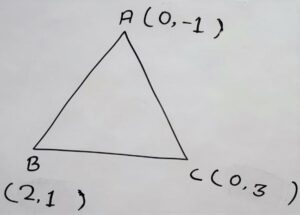According to diagram

co-ordinates of :

A = (0,-1)

B = (2,1)

C = (0,3)

Mid-point of AB is D

Co-ordinate of D

x = (x1+x2)/2 ; y = (y1+y2)/2  [By mid-point formula]

⇒ x = (0+2)/2 ; y = (-1+1)/2

⇒ x = 1 ; y = (0)/2

⇒ x = 1 ; y = 0

D = (1,0)

Mid-point of BC is E

Co-ordinate of E

x = (x1+x2)/2 ; y =  (y1+y2)/2  [By mid-point formula]

⇒ x = (2+0)/2 ; y = (1+3)/2

⇒ x = 2/2 ; y = 4/2

= x = 1 ; y = 2

E = (1,2)

Mid-point of AC is F

Co-ordinate of F

x = (x1+x2)/2 ; y = (y1+y2)/2  [By mid-point formula]

⇒ x = (0+0)/2 ; y = (-1+3)/2

⇒ x = 0/2 ; y = 2/2

⇒ x = 0 ; y = 1

F (0,1)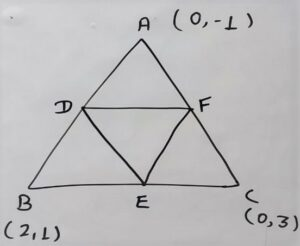For the area of the triangle DEF

D = (1,0), E = (1,2), F (0,1)

x1 = 1 ; y1 = 0

x2 = 1 ; y2 = 2

x3 = 0 ; y3 = 1

Area of ΔDEF = 1/2 [ x1 (y2 – y3) + x2 (y3 – y1) + x3 (y1 – y2) ]

⇒ Area of ΔDEF = 1/2 [ 1 (2 – 1) + 1 (1 – 0) + 0 (0 – 2) ]

⇒ Area of ΔDEF = 1/2 [ 1 (1) + 1 (1) + 0 (- 2) ]

⇒ Area of ΔDEF = 1/2 [ 1 + 1 + 0 ]

⇒ Area of ΔDEF = 1/2 [ 2]

⇒ Area of ΔDEF = 1 square unit

Here

x1 = 0 ; y1 = -1

x2 = 2 ; y2 = 1

x3 = 0 ; y3 = 3

Area of Δ ABC = 1/2 [ x1 (y2 – y3) + x2 (y3 – y1) + x3 (y1 – y2) ]

⇒ Area of Δ ABC = 1/2 [ 0 (1 – 3)  + 2 (3 – {-1}) + 0 (-1 – 1) ]

⇒ Area of Δ ABC = 1/2 [ 0 (-2)  + 2 (3 +1) + 0 (-2) ]

⇒ Area of Δ ABC = 1/2 [ 0 + 2 (4) + 0 ]

⇒ Area of Δ ABC = 1/2 [ 0 + 8 + 0 ]

⇒ Area of Δ ABC = 1/2 [ 8 ]

⇒ Area of Δ ABC = 4 square units

For Ratio

Area of Δ DEF÷ Area of Δ ABC = 1÷4

⇒ Area of Δ DEF: Area of Δ ABC = 1:4

Q.4 Find the area of the quadrilateral whose vertices, taken in order, are (-4,-2),(-3,-5),(3,-2), and (2,3).

Solution:

Let Quadrilateral ABCD whose Co-ordinates are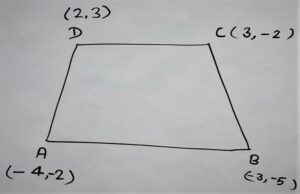A(-4,-2)

B(-3,-5)

C( 3,-2)

D(2,3)

After joining diagonal AC we get two triangles ΔABC and ΔACD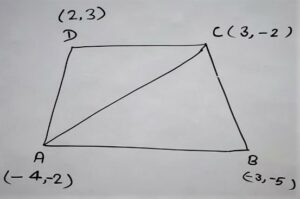∴ Area of Quadrilateral ABCD = Area ABC + Area ACD

In Δ ABC

A(-4,-2)

B(-3,-5)

C( 3,-2)

x1 = -4 ; y1 = -2

x2 = -3 ; y2 = -5

x3 = 3 ; y3 = -2

Area of Δ ABC = 1/2 [ x1 (y2 – y3) + x2 (y3 – y1) + x3 (y1 – y2) ]

⇒ Area of Δ ABC = 1/2 [ -4(-5 +2) -3 (-2 + 2) + 3 (-2 + 5) ]

⇒ Area of Δ ABC = 1/2 [ -4(-3) -3 (0) + 3 (3) ]

⇒ Area of Δ ABC = 1/2 [ 12 + 0 + 9 ]

⇒ Area of Δ ABC = 1/2 [ 21 ]

⇒ Area of Δ ABC = 21/2 square units

In Δ ACD

A(-4,-2)

C( 3,-2)

D(2,3)

x1 = -4 ; y1 = -2

x2 = -3 ; y2 = -5

x3 = 2 ; y3 = -3

Area of Δ ACD = 1/2 [ x1 (y2 – y3) + x2 (y3 – y1) + x3 (y1 – y2) ]

⇒ Area of Δ ACD = 1/2 [ -4 (-5 + 3 ) – 3 (-3 + 2) + 2 (-2 + 5) ]

⇒ Area of Δ ACD = 1/2 [ -4 (-2 ) – 3 (-1) + 2 (3) ]

⇒ Area of Δ ACD = 1/2 [ 8 + 3 + 6 ]

⇒ Area of Δ ACD = 1/2 

⇒ Area of Δ ACD = 17/2

Area of Quadrilateral ABCD = Area ABC + Area ACD

⇒ Area of Quadrilateral ABCD = 21/2 + 17/2

⇒ Area of Quadrilateral ABCD = 38/2

⇒ Area of Quadrilateral ABCD = 19 Square units

Q.5 You have studied in Class 9th,(Chapter 9, Example 3), that a median of a triangle divides it into two triangles of equal areas. Verify this result for Δ ABC whose vertices are A(4, -6), B(3,-2), and C(5,2).

Solution: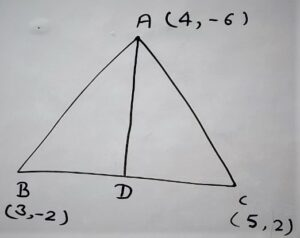In Δ ABC

From vertex A drawn a median AD on side BC.

Here D is midpoint of B(3,-2) and C(5,2)

By mid-point formula

Co-ordinate of D :

x = (x1+x2)/2 ; y =  (y1+y2)/2  [By mid-point formula]

⇒ x = (3+5)/2 ; y = (-2+2)/2

⇒ x = 8/2 ; y = 0/2

⇒ x = 4 ; y = 0

D = (4,0)

A = (4,-6)

D = (4,0)

B = (3,-2)

Area of ΔADB = 1/2 [ x1 (y2 – y3) + x2 (y3 – y1) + x3 (y1 – y2) ]

⇒ Area of ΔADB = 1/2 [ 4 (0 + 2) + 4 (-2 + 6) + 3 (-6 – 0) ]

⇒ Area of ΔADB = 1/2 [ 4 (2) + 4 (4) + 3 (-6 ) ]

⇒ Area of ΔADB = 1/2 [ 8 + 16 – 18 ]

⇒ Area of ΔADB = 1/2 

⇒ Area of ΔADB = 3 square units

A = (4,-6)

D = (4,0)

C = (5,2)

Area of ΔADC = 1/2 [ x1 (y2 – y3) + x2 (y3 – y1) + x3 (y1 – y2) ]

⇒ Area of ΔADC = 1/2 [ 4(0 – 2) + 4 (2 + 6) + 5 (-6 – 0) ]

⇒ Area of ΔADC = 1/2 [ 4(- 2) + 4 (8) + 5 (-6) ]

⇒ Area of ΔADC = 1/2 [ -8 + 32 -30 ]

⇒ Area of ΔADC = 1/2 [ -38+ 32 ]

⇒ Area of ΔADC = 1/2 [ -6 ]

⇒ Area of ΔADC = -3 (area never be negative)

⇒ Area of ΔADC = 3square units

Hence

Area of ΔABC = Area of ΔACD